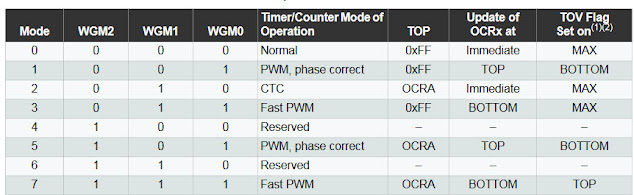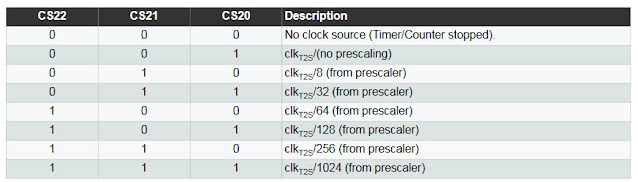# Time Delay with Arduino Timer 2

Here it is shown how to write time delay routine with Timer 2 of Arduino. Time delay is required in many programs to create wait time for example in LED blink, waiting for user inputs, waiting for sensor input etc. There are time delay function in Arduino such as delay(), milli() and micro() but they are tied to Timer 0 and sometimes you need Timer 0 function for other task such as counting external events. Also many libraries uses Timer 0 so when you need Timer 0 for other task and you need delay function it is good to learn how to program time delay with other timer such as timer 1 or timer 2.

To create the time delay using timer 2 we need to know how the timer 2 works and know the registers associated with it. The following shows the timer 2 block diagram of Arduino which is based on ATmega328p microcontroller.

When timer/counter 2 is used as a time delay timer we operate it in normal mode. To operate the timer in normal mode we have to set the appropriate bits in the TCCR2(TCCR2A,TCCR2B) registers.

The TCCR2 registers is shown below.

The waveform generation mode bits WGM22, WGM21, WGM20 defines mode of operation which are all zero for normal mode.The timer 2 clock select bits C22, C21 and CS20 are required here whose bit combination gives different pre-scalar values.So for normal mode with 1024 prescalar the setting for TCCR0A and TCCR2B registers are:

TCCR2A = 0x00;

TCCR2B = 0b00000111;

In normal mode, the timer 2 TCNT2 register is loaded with count value. The count value is the value that when counted to from zero gives the required time delay.

The formula to calculate the count value to be loaded in the TCNT2 register for specified time delay(Td) is given by,

$$C = 256 -\frac{ F_{CPU}}{NF_d}$$

If for example we want time delay($$T_d$$) of 1ms, if the CPU or the oscillator frequency($$F_{CPU}$$) is 8MHz and if we have prescalar of 1024 then,

$$C = 256 -\frac{ F_{CPU}}{NF_d}= 256 - \frac{8MHz}{1024 \times 1KHz} = 256 - 8 =248$$

where, $$F_d=\frac{1}{T_d}=\frac{1}{1ms}=1KHz$$

So we have to load TCNT2 register with count value C=248.

After the TCNT2 is loaded with count value, the timer is started and the timer counts from zero to the count value. When it reaches the count value, the overflow flag TOV2 which resides in TIFR2 is set. We have to monitor this TOV2 flag and stop the timer in software and restart the timer. The TIFR2 register is shown below.

The following is function for 1ms time delay with timer 2 for Arduino.


void T2delayOnems(){
//setup Timer 2
TCNT2 = 248;		//load count value for 1ms time delay
TCCR2A = 0x00;
TCCR2B = 0b00000111;	 //normal mode with 1024 pre-scalar

while(!(TIFR2 & (1<<TOV2)));	//wait until TOV0 flag is set
TCCR2B = 0;		//turn off timer 0
TIFR2 |= (1<<TOV2);	//clear TOV0 flag
}


In the above code, we load the count value of 248 into the TCNT2 register. We initialize the Timer 2 in normal mode and with pre-scalar of 1024 by configuring the TCCR02 registers. Then we use while loop function to monitor the TOV2 flag and when it is set we turn off the timer and then we clear the TOV2 flag by setting it high.

Another function can be created that calls the above 1ms function to create a delay of required number of milli second.


void T2delayms(int n){
for(int i = 0; i <= n; i++){
T2delayOnems();
}
}


The following is program code to blink a LED with 100ms time delay that uses the above two time delay functions.


#define LED PD3

void T2delayOnems(void);
void T2delayms(int n);

void setup(){
pinMode(LED,OUTPUT);
}

void loop(){
digitalWrite(LED, HIGH);
T2delayms(100);
digitalWrite(LED, LOW);
T2delayms(100);
}

void T2delayOnems(){
//setup Timer 2
TCNT2 = 248;		//load count value for 1ms time delay
TCCR2A = 0x00;
TCCR2B = 0b00000111;	 //normal mode with 1024 pre-scalar

while(!(TIFR2 & (1<<TOV2)));	//wait until TOV2 flag is set
TCCR2B = 0;		//turn off timer 0
TIFR2 |= (1<<TOV2);	//clear TOV0 flag
}

void T2delayms(int n){
for(int i = 0; i <= n; i++){
T2delayOnems();
}
}


So in this way we can create required time delay with Arduino Timer 2. For Timer 0 time delay programming see the previous tutorial Time delay using timer 0 without inbuild functions in Arduino.